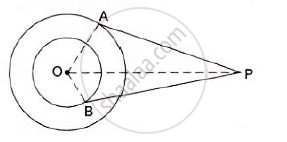# In the Given Figure, O is the Centre of the Two Concentric Circles of Radii 4 Cm and 6cm Respectively. If Pa = 10cm, Find the Length of Pb up to One Place of the Decimal.. - Mathematics

In the given figure, O is the centre of the two concentric circles of radii 4 cm and 6cm respectively. AP and PB are tangents to the outer and inner circle respectively. If PA = 10cm, find the length of PB up to one place of the decimal.#### Solution

Given, O is the center of two concentric circles of radii OA = 6 cm and OB = 4 cm.
PA and PB are the two tangents to the outer and inner circles respectively and PA = 10 cm.
Now, tangent drawn from an external point is perpendicular to the radius at the point of contact.
∴∠OAP = ∠OBP = 90°
∴ From right – angled ΔOAP,OP2  = OA+  PA2

⇒OP = sqrt(OA^2 + PA^2)

⇒ OP = sqrt (6^2 +10^2)

⇒ OP = sqrt( 136cm).

∴ From right – angled Δ OAP,OP^2 = OB^2 + PB^2

⇒ PB = sqrt(OP^2 -OB^2)

⇒ PB = sqrt(136-16)

⇒ PB= sqrt(120cm)

⇒ PB = 109.cm.

∴ The length of PB is 10.9 cm.

Concept: Concept of Circle - Centre, Radius, Diameter, Arc, Sector, Chord, Segment, Semicircle, Circumference, Interior and Exterior, Concentric Circles
Is there an error in this question or solution?